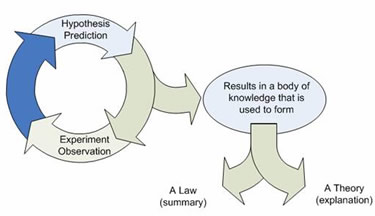# Difference between Hypothesis and Theory

##### Key Difference: A Hypothesis is a tentative statement which provides explanations regarding a phenomenon or event. It is widely used as a base for conducting tests and the results of the tests determine the acceptance or rejection of the hypothesis. A Theory is the scientific explanation of an observed activity or a phenomenon. It explains the observations or events that are based upon any proven hypothesis. Therefore, hypothesis forms the base for a theory.A Hypothesis is an uncertain explanation regarding a phenomenon or event. It is widely used as a base for conducting tests and the results of the tests determine the acceptance or rejection of the hypothesis. A tentative statement takes a form like - “If X happens then Y must happen”. Hypothesis can be tested by various methods, including the direct experimentation or using certain observation skills. It is important to mention that theories, laws and facts can start from being just a hypothesis at the initial phase.

It can always be tested by experimentation after the formulation. It is generated by gathering possible evidence. Later, the investigation is carried out to test the hypothesis. It is either accepted or assumed.

A Theory is basically a system comprising of ideas through which explanations are made. A theory is based on the hypothesis. If the hypothesis is able to survive and pass all critical tests then it is eligible to be mentioned as a theory. A theory is always supported by evidence. Therefore, it is regarded to be a well confirmed type of explanation. A theory may also be used for predicting any of the future observations. One of the famous theories is Darwin’s Revolutionary Theory. Darwin derived and used the information from various fields and subjects to establish this theory.

Hypothesis and theory are related to each other but still differ in many aspects. Hypothesis is a testable proposition, whereas theory is a coherent set of propositions. Hypothesis is always testable and this property defines the very basic property of a hypothesis. On the other hand, theory is a result that is obtained by rigorous testing and investigations.

Comparison between Hypothesis and Prediction:

 Hypothesis Theory Definition A Hypothesis is an uncertain explanation regarding a phenomenon or event. It is widely used as a base for conducting tests and the results of the tests determine the acceptance or rejection of the hypothesis. Theory is basically a system comprising of ideas through which explanations are made. A theory is based on the hypothesis. If the hypothesis is able to survive and pass all critical tests then it is eligible to be mentioned as a theory. Origin The term derived from the Greek, hypotithenai meaning "to put under" or "to suppose." The term derived from Greek theōria 'contemplation, speculation', from theōros 'spectator.' Proving methodology Various experiments can lead to various results. Thus, a hypothesis can be proven or rejected depending upon the method used by the scientists. It summarizes a hypothesis or group of hypotheses which have been supported by repeated testing. It remains valid till the evidence is determined to oppose it. Supported by Reasoning Yes Completely Example Ultra violet light may cause skin cancer. The theory of evolution. The heliocentric theory of the solar system. Importance A proven hypothesis can lead to a law or a theory. Very broad applications and explanatory power.

Image Courtesy: freethinkerperspective.blogspot.com